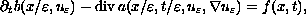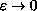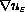Electron. J. Diff. Eqns., Vol. 2001(2001), No. 17, pp. 1-19.

### Homogenization of a nonlinear degenerate parabolic differential equation A. K. Nandakumaran & M. Rajesh

Abstract:
In this article, we study the homogenization of the nonlinear degenerate parabolic equationwith mixed boundary conditions(Neumann and Dirichlet) and obtain the limit equation as. We also prove corrector results to improve the weak convergence ofto strong convergence.

Submitted September 11, 2000. Published March 15, 2001.
Math Subject Classifications: 35B27, 74Q10.
Key Words: degenerate parabolic equation, homogenization, two-scale convergence, correctors.

Show me the PDF file (292K), TEX file, and other files for this article.A. K. Nandakumaran Department of Mathematics Indian Institute of Science Bangalore- 560 012, India e-mail: nands@math.iisc.ernet.in M. Rajesh Department of Mathematics Indian Institute of Science Bangalore- 560 012, India e-mail: rajesh@math.iisc.ernet.in